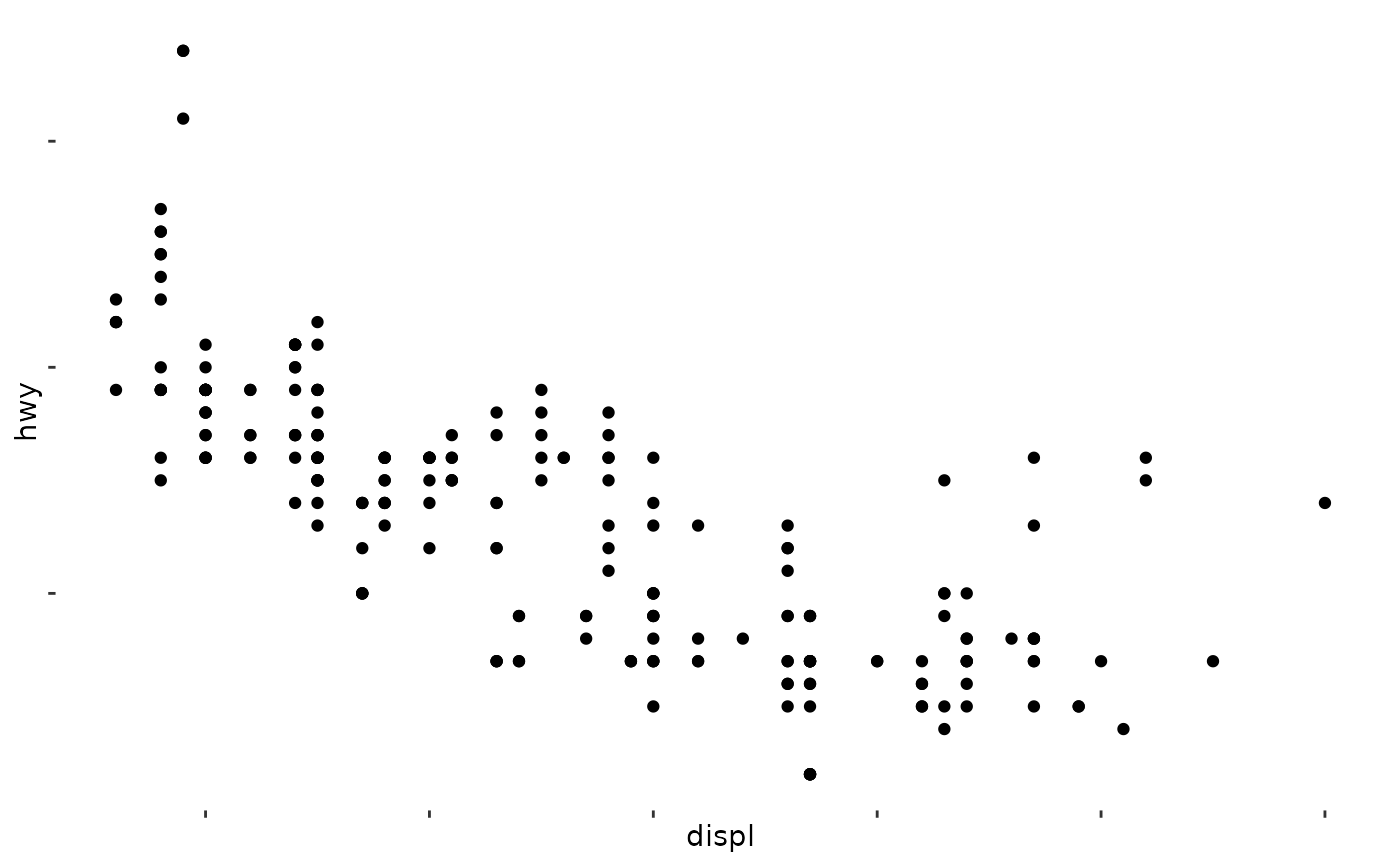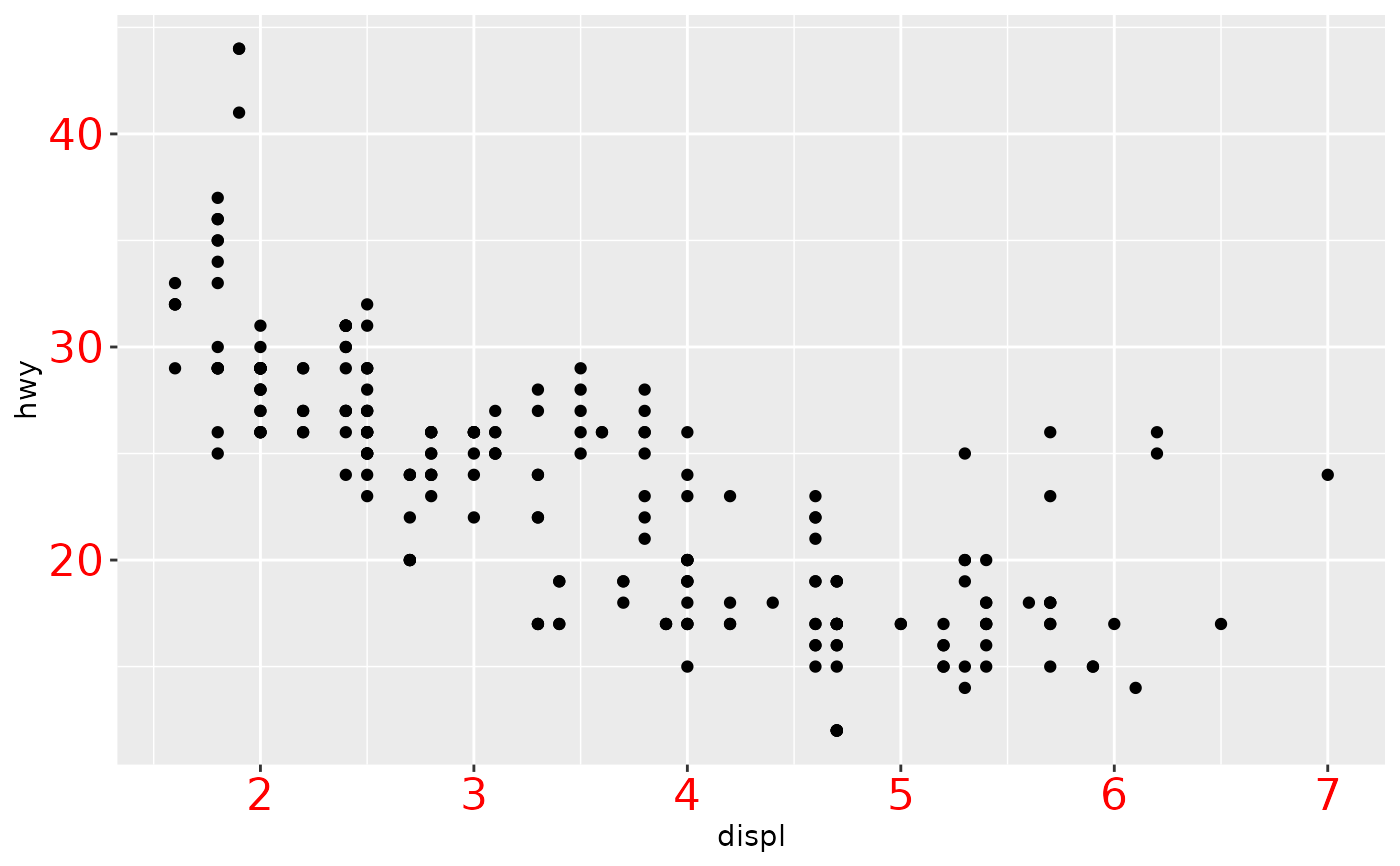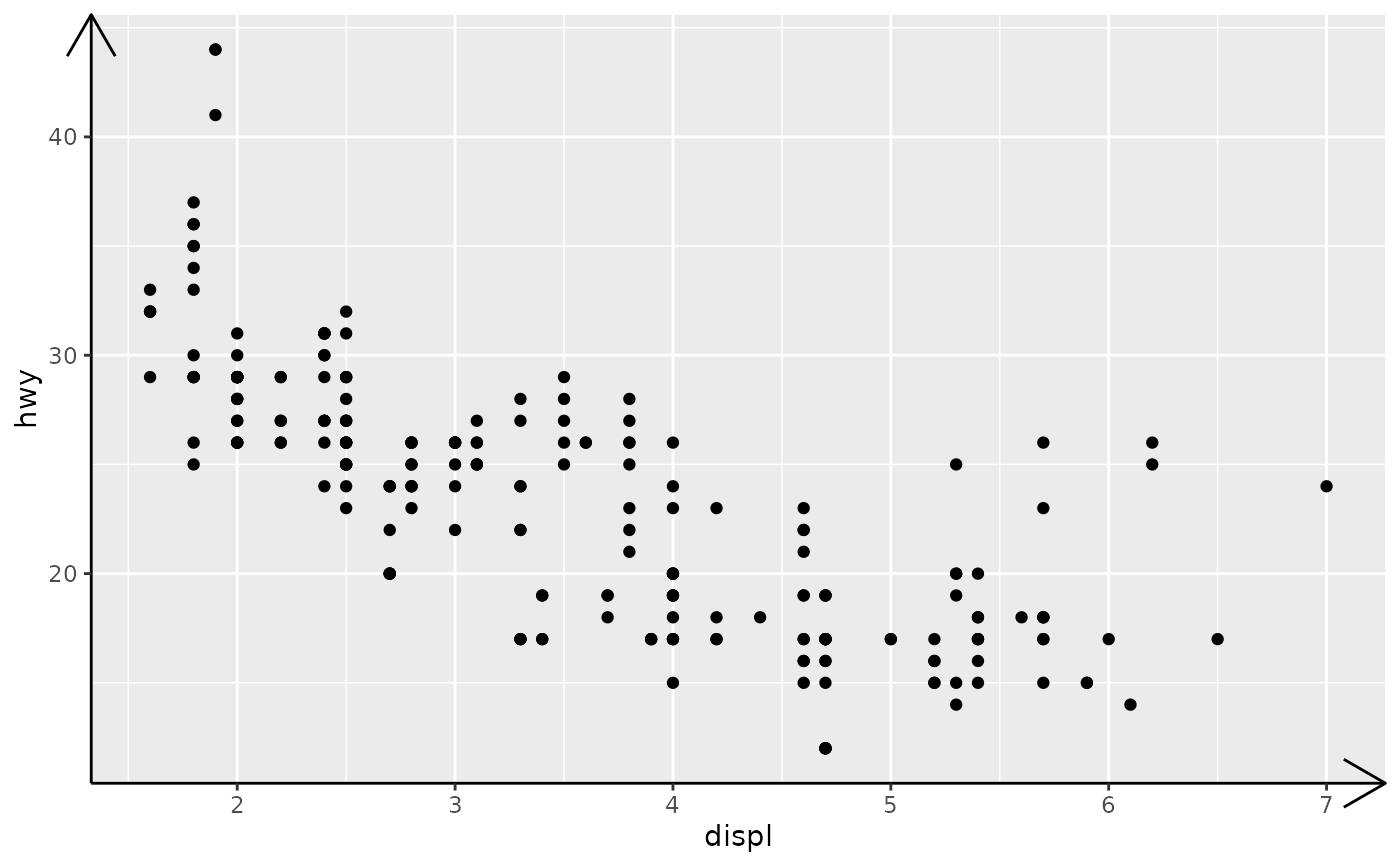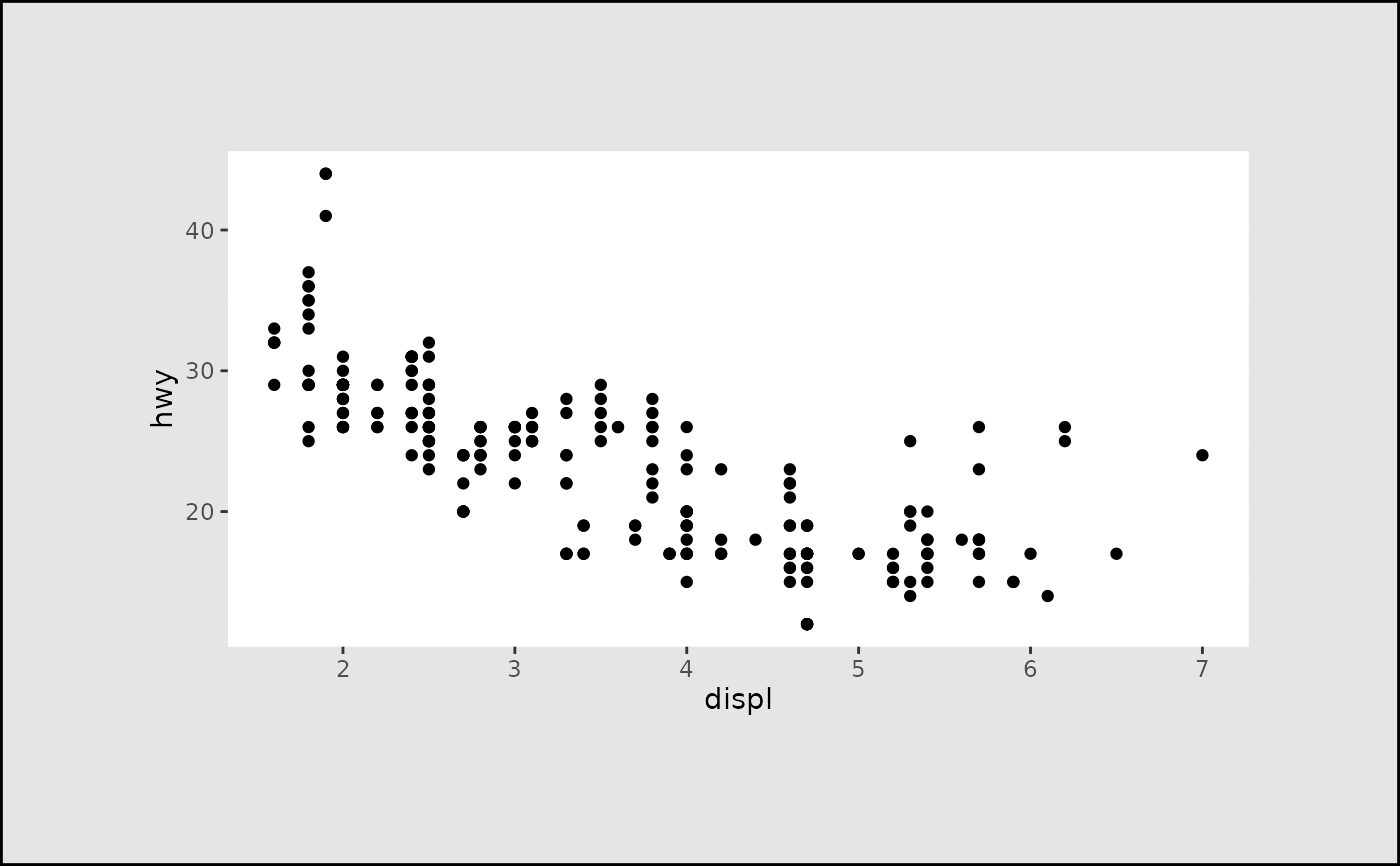In conjunction with the theme system, the element_ functions specify the display of how non-data components of the plot are drawn.

• element_blank(): draws nothing, and assigns no space.

• element_rect(): borders and backgrounds.

• element_line(): lines.

• element_text(): text.

rel() is used to specify sizes relative to the parent, margin() is used to specify the margins of elements.

## Usage

margin(t = 0, r = 0, b = 0, l = 0, unit = "pt")

element_blank()

element_rect(
fill = NULL,
colour = NULL,
linewidth = NULL,
linetype = NULL,
color = NULL,
inherit.blank = FALSE,
size = deprecated()
)

element_line(
colour = NULL,
linewidth = NULL,
linetype = NULL,
lineend = NULL,
color = NULL,
arrow = NULL,
inherit.blank = FALSE,
size = deprecated()
)

element_text(
family = NULL,
face = NULL,
colour = NULL,
size = NULL,
hjust = NULL,
vjust = NULL,
angle = NULL,
lineheight = NULL,
color = NULL,
margin = NULL,
debug = NULL,
inherit.blank = FALSE
)

rel(x)

## Arguments

t, r, b, l

Dimensions of each margin. (To remember order, think trouble).

unit

Default units of dimensions. Defaults to "pt" so it can be most easily scaled with the text.

fill

Fill colour.

colour, color

Line/border colour. Color is an alias for colour.

linewidth

Line/border size in mm.

linetype

Line type. An integer (0:8), a name (blank, solid, dashed, dotted, dotdash, longdash, twodash), or a string with an even number (up to eight) of hexadecimal digits which give the lengths in consecutive positions in the string.

inherit.blank

Should this element inherit the existence of an element_blank among its parents? If TRUE the existence of a blank element among its parents will cause this element to be blank as well. If FALSE any blank parent element will be ignored when calculating final element state.

size

text size in pts.

lineend

Line end Line end style (round, butt, square)

arrow

Arrow specification, as created by grid::arrow()

family

Font family

face

Font face ("plain", "italic", "bold", "bold.italic")

hjust

Horizontal justification (in $$[0, 1]$$)

vjust

Vertical justification (in $$[0, 1]$$)

angle

Angle (in $$[0, 360]$$)

lineheight

Line height

margin

Margins around the text. See margin() for more details. When creating a theme, the margins should be placed on the side of the text facing towards the center of the plot.

debug

If TRUE, aids visual debugging by drawing a solid rectangle behind the complete text area, and a point where each label is anchored.

x

A single number specifying size relative to parent element.

## Value

An S3 object of class element, rel, or margin.

## Examples

plot <- ggplot(mpg, aes(displ, hwy)) + geom_point()

plot + theme(
panel.background = element_blank(),
axis.text = element_blank()
)plot + theme(
axis.text = element_text(colour = "red", size = rel(1.5))
)plot + theme(
axis.line = element_line(arrow = arrow())
)plot + theme(
panel.background = element_rect(fill = "white"),
plot.margin = margin(2, 2, 2, 2, "cm"),
plot.background = element_rect(
fill = "grey90",
colour = "black",
linewidth = 1
)
)